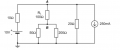# Thevenin Eqivalent circuit

#### Matthew Zammit

Joined Jan 14, 2016
2
How can i find the thevenin equivalent circuit for RL?
I converted the current source to a 5v source inseries with the 20ohm resistor but i always seem to get stuck when i have more than one source. I used superposition and got an Itotal of 0.5amps... whats next? Do the 50 and 150ohm resistors some into play or are they considered as a short circuit? Thanks in advance.Last edited:

#### Matthew Zammit

Joined Jan 14, 2016
2
Update I got an answer of 5v vth and 46.67ohms rth. Correct or not?

#### WBahn

Joined Mar 31, 2012
28,486
One nice thing about circuit problems (most engineering problems in general) is that you can usually verify the validity of a proposed answer directly from the problem itself, often in multiple ways.

Use your Thevenin circuit circuit to find the current through RL. Then use the now known current in RL to find the current through the 50 Ω and 200 Ω resistors (they are a current divider, so that should be easy to do). Now you can find the voltage between the top and bottom rails, which let you find the currents in the 10 Ω and 20 Ω resistors very easily. Now sum up the current in these three branches and see if it is consistent with the 250 mA source.

But before you do that, you might do some sanity checks on your answer.

For instance, if you remove RL then there will be no current in the resistors below it, so Node B will have the same voltage as the bottom rail. Due to the polarity of the current source, when you convert it to a voltage source the left side will be at -10 V and the right side at -5 V. With the larger resistor to the right, that will mean that the voltage at Node A will be closer to the -5 V than it will be to +10 V, so you know the open circuit voltage (unless I've missed something by doing all this in my head, which is quite possible) will be less than 2.5 V and might well be negative.

Another sanity check is to consider the bounds on the equivalent resistance. After zeroing both the sources, you have two parallel paths from Node A to the bottom rail and then two parallel paths from the bottom rail up to Node B. The resistance of two parallel resistors can be no less than half the smallest resistor and no more than the smallest resistor. So the first path has to be between 5 Ω and 10 Ω while the second path has to be between 25 Ω and 50 Ω. Thus the equivalent resistance must lie between 30 Ω and 60 Ω. So you pass this check, which isn't sufficient to know the answer is correct, but it is necessary for the answer to be correct.